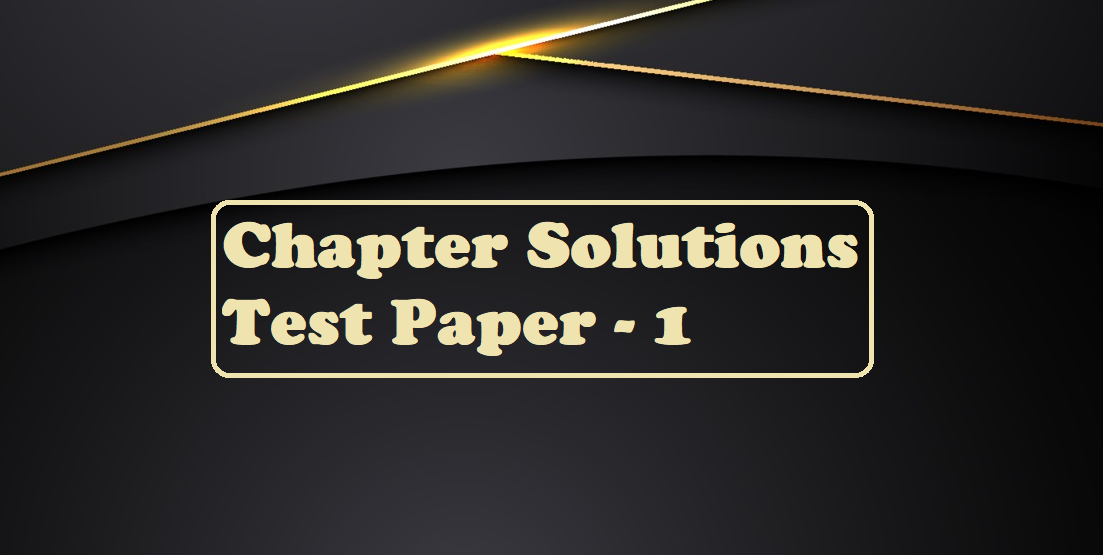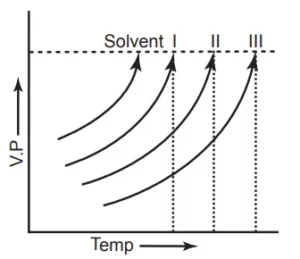# Chapter Solutions Test Paper – 1# Chapter Solutions Test Paper – 1

Chapter Solutions Test Paper

Q 1. Concentrated nitric acid used in the laboratory work is 68% nitric acid by mass in an aqueous solution. What should be the molarity of such a sample of the acid if the density of the solution is 1.504 g mL–1?
(A) 12.12 mol L–1
(B) 20.12 mol L–1
(C) 16.23 mol L–1
(D) 34.78 mol L–1

Q 2. A sample of drinking water was found to be severely contaminated with chloroform, CHCl3, supposed to be a carcinogen. The level of contamination was 15 ppm (by mass). Determine the molality of chloroform in the water sample.
(A) 2.12 × 10–4 mol kg–1
(B) 1.26 × 10–4 mol kg–1
(C) 0.12 × 10–4 mol kg–1
(D) 5.34 × 10–4 mol kg–1

Q 3. An aqueous solution of hydrochloric acid-
(A) Obeys Raoult’s law.
(B) Shows negative deviations from Raoults’ law.
(C) Shows positive deviations from Raoults’ law.
(D) Obeys Henrys’ law at all compositions.

Q 4. The vapour pressure of a dilute aqueous solution of glucose (C6H12O6) is p atm. at 373K. The mole fraction of the solvent is
(A) P atm.
(B) (P0 – P) atm.
(C) P0 atm.
(D) (P0 – P)/ P0 atm.

Q 5. The vapour pressure of pure toluene and n-heptane at 50°C are 92.6 mm Hg and 140.9mm Hg. The composition of the liquid mixture of toluene, A, and n-heptane, B at 50°C having PA = PB is
(A) 0.41-mole fraction of A
(B) 0.522-mole fraction of A
(C) 0.603-mole fraction of A
(D) 0.373-mole fraction of A

Q 6. Which of the following quantities is independent of temperature?
(A) Molarity
(B) Normality
(C) Molality
(D) Formality

Q 7. Which of the following colligative properties is associated with the concentration term ‘molarity’?
(A) Lowering of vapour pressure.
(B) Osmotic pressure.
(C) Depression in freezing point.
(D) Elevation in boiling point.

Q 8. The Vapour pressure of water at 293 K is 17.535 mm Hg. Calculate the vapour pressure of water at 293 K when 25g of glucose is dissolved in 450 g of water.
(A) 17.44 mm Hg
(B) 12.12 mm Hg
(C) 20.56 mm Hg
(D) 26.12 mm Hg

Q 9. The mole fraction of urea (Mol. wt. = 60)  in a 1.0 molal ethanolic solution of the compound is-
(A) 0.145
(B) 0.064
(C) 0.044
(D) 0.290

Q.10   The vapour pressure of  CHCl3 and CCl4 are 280 and 200mm Hg at 27°C. Calculate the mole fraction of each in the vapour phase when a mixture containing 2 moles of CHCl3 and  8moles of CCl4 is maintained at 27°C.
(A) 0.26, 0.741
(B) 0.31, 0.69
(C) 0.78, 0.22
(D) 0.5, 0.5

Q 11. A 5% solution (by mass) of cane sugar in water has a freezing point of 271 K. Calculate the freezing point of a 5 % glucose in water if the freezing point of pure water is 273.15 K.
(A) 269.07 K
(B) 230.27 K
(C) 290.78 K
(D) 302.02 K

Q 12. The osmotic pressure of equimolar solutions of BaCl2, NaCl and glucose will be in the order –
(A)BaCl2 > NaCl > glucose
(B) Glucose > NaCl > BaCl2
(C) NaCl > BaCl2 > Glucose
(D) NaCl > Glucose > BaCl2

Q 13. Which of the following aqueous solution has osmotic pressure nearest to that of an equimolar solution of K4[Fe(CN)6]?
(A) Na2SO4
(B) BaCl2
(C) Al2(SO4)3
(D) C12H22O11

Q.14   Calculate the mass of a solvent in the mixture obtained by mixing 300 g of a 25% and 400 g of a 40% solution by mass.
(A) 66.4%
(B) 55.2%
(C) 72.1%
(D) 58.2%

Q 15. A 25 gm cube of ice at 0 0C is added to 100 gm of liquid at 22.0 0C in a thermally insulated container. The heat of fusion of ice is 6.01 KJ mol–1 and the specific heat of liquid water is 4.18 J gm–1 0C–1. What is the temperature in the final condition?
(A) 1.625 0C
(B) 0.625 0C
(C) 3.125 0C
(D) 2.635 0C

Chapter Solutions Test Paper

Q 16. Acetone has a vapour pressure of 185.2 mm Hg and ether 443.5 mm Hg at 20ºC. For a liquid mixture of acetone and ether containing 0.457-mole fraction of acetone, the partial pressure of ether is
(A) 218.8 mm
(B) 240.8 mm
(C) 318.8 mm
(D) 381.8 mm

Q 17. A dilute solution may be defined as one in which
(A) The solvent obeys Raoult’s law.
(B) The solution has a higher boiling point than the pure solvent.
(C) The solution has a lower vapour pressure than the pure solvent.
(D) The solution has a lower freezing point.

Q 18. Which of these aqueous solutions would have the lowest freezing point?
(A) 1.0 molal glucose sugar
(B) 1.0 molal magnesium sulphate
(C) 1.0 molal caesium iodide
(D) 1.0 molal lithium sulphate

Q 19. The osmotic pressure of an aqueous solution of sucrose at 303 K is 2.47 atm, and the molar volume of water at this temperature is 18.10 cm3. Calculate the elevation of the boiling point of this solution DH vap = 539 cal gm–1.
(A) 0.51 K
(B) 0.12 K
(C) 0.051 K
(D) 0.721 K

Q 20. Concentrated aqueous sulphuric acid is 98% H2SO4 by mass and has a density of 1.80 g /ml. The volume of acid required to make one litre of 0.1 M H2SO4 is
(A) 5.55 ml
(B) 11.10 ml
(C ) 16.65 ml
(D) 22.20 ml

Q 21. An aqueous solution is 1.00 molal in KI. Which change will cause the vapour pressure of the solution to increase?
(A) Addition of 1.00 molal KI

Q 22. 6% (W/V) solution of urea will be isotonic with:
(A) 18% (W/V) solution of glucose
(B) 0.5 M solution of NaCl
(C) 1 M solution of CH3COOH
(D) 6% (W/V) solution of sucrose.

Q 23. The aqueous solution that has the lowest vapour pressure at a given temperature is:
(A) 0.1 molal sodium phosphate
(B) 0.1 molal barium chloride
(C) 0.1 molal sodium chloride
(D) 0.1 molal glucose

Q 24. The vapour pressure curves of a solute in a solvent are shown below. These are not only parallel but also do not intersect one anotherWhich is the correct order of concentration of solutions here?
(a) 1 > 2 > 3
(b) 3 > 2 > 1
(c) 2 > 3 > 1
(d) 1 > 3 > 2

Chapter Solutions Test Paper